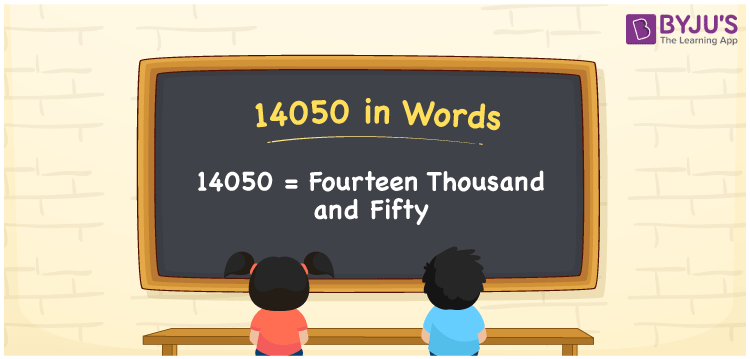# 14050 in Words

The number name of 14050 is “Fourteen Thousand and Fifty”. For example, if you spent Rs. 14050 in 2 months, you can write it as “I spent Rs. Fourteen Thousand and Fifty in two months”. Hence, for converting the number 14050 into words, the place value system is useful. This article will help you to learn the procedure of writing the number 14050 in words with a detailed explanation.

 14050 in Words: Fourteen Thousand and Fifty. Fourteen Thousand and Fifty in Numerical Form: 14050.

## 14050 in English Words## How to Write 14050 in Words?

As discussed above, the place value system is found to be helpful while writing 14050 in words. The below table presents the place values of the number 14050.

 Ten-thousands Thousands Hundreds Tens Ones 1 4 0 5 0

The expanded form of 14050 is as follows:

= 1 × Ten thousand + 4 × Thousand + 0 × Hundred + 5 × Ten + 0 × One

= 1 × 10000 + 4 × 1000 + 0 × 100 + 5 × 10 + 0 × One

= 10000 + 4000 + 50

= 14050

= fourteen thousand and fifty

Hence, 14050 in words is fourteen thousand and fifty.

The interesting facts about the number 14050 are given below:

14050 in words – Fourteen thousand and fifty

Is 14050 an odd number? – No

Is 14050 an even number? – Yes

Is 14050 a perfect square number? – No

Is 14050 a perfect cube number? – No

Is 14050 a prime number? – No

Is 14050 a composite number? – Yes

## Frequently Asked Questions on 14050 in Words

### Write 14050 in words.

14050 in words is fourteen thousand and fifty.

### Simplify 14000 + 50, and express it in words.

Simplifying 14000 + 50, we get 14050. Hence, 14050 in words is fourteen thousand and fifty.

### Is 14050 a composite number?

Yes, 14050 is a composite number.